• 本篇文章给大家带来的内容是关于Python绘制折线图散点图的详细方法介绍（代码示例），有一定的参考价值，有需要的朋友可以参考一下，希望对你有所帮助。1、绘制折线图散点图要用到matplotlib下的pylab，因此我们...
本篇文章给大家带来的内容是关于Python绘制折线图和散点图的详细方法介绍（代码示例），有一定的参考价值，有需要的朋友可以参考一下，希望对你有所帮助。
1、绘制折线图和散点图要用到matplotlib下的pylab，因此我们需要先引入，因为要用到数组实现，还要引入numpy。然后确定x轴和y轴的数据，最后将其呈现出来。import matplotlib.pylab as pyl
import numpy as np
x = [1, 3, 5, 6, 8, 13, 14, 16]
y = [5, 1, 6, 7, 9, 3, 2, 10]
pyl.plot(x, y)
pyl.show()
这样一个简单的折线图就绘制好了。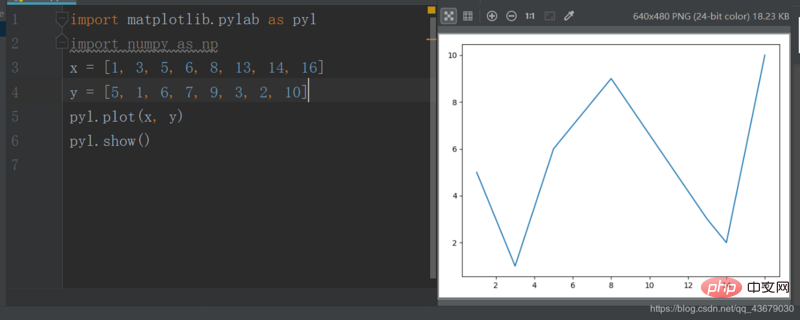上面的plot()有三个参数，第一个参数为x轴坐标，第二个参数为y轴坐标，第三个参数为确定线型，可有可无，如果要将上面的折线图改为散点图，只需更改第三个参数为"o’。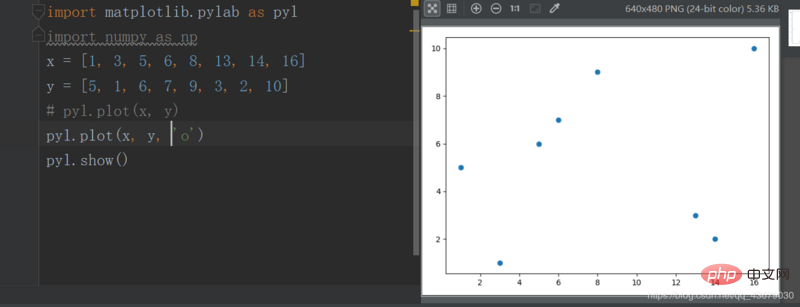如果让折线图和散点图叠加还可以突出每个点。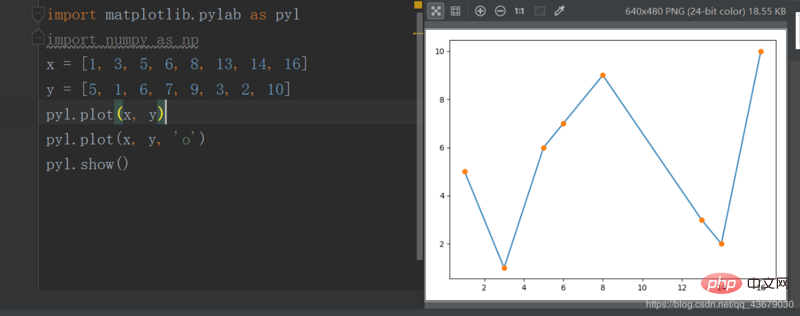2.我们还可以改变线和点的颜色，只需修改plot()的第三个参数。c--cyan--青色
r--red--红色
m--magente--品红
g--green--绿色
b--blue--蓝色
y--yellow--黄色
k--black--黑色
w--white--白色
上述参数可以叠加。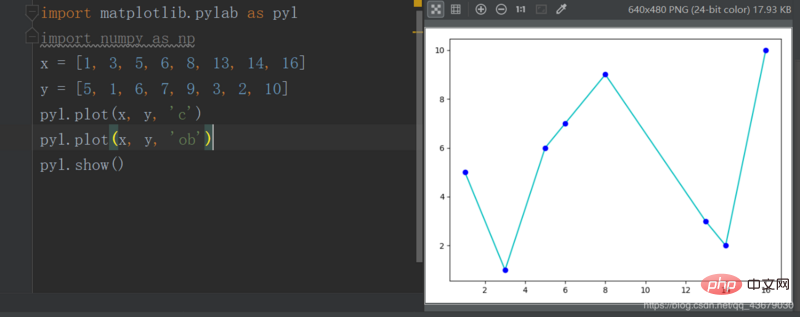3.我们还可以改变线型，也是修改plot的第三个参数。- 实线
-- 虚线
-. 形式即为-.
: 细小的虚线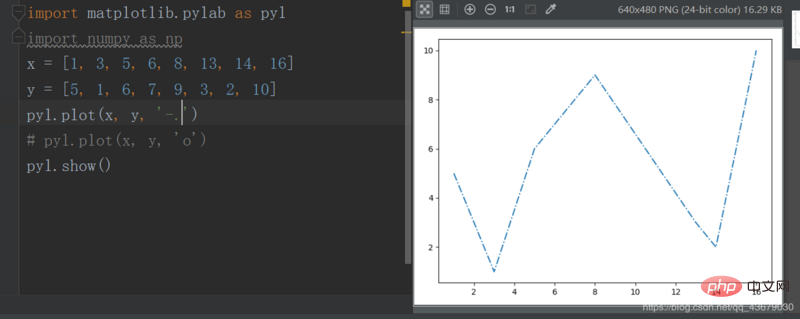4.我们还可以改变点型，同样是修改第三个参数。s--方形
h--六角形
H--六角形
*--*形
+--加号
x--x形
d--菱形
D--菱形
p--五角形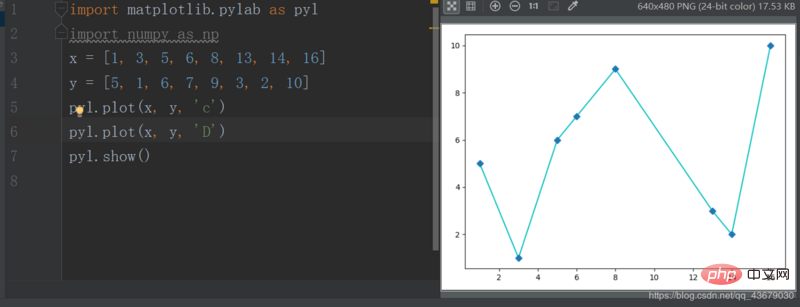5.我们目前绘制的图形，无图像名称及横纵坐标轴的名称，我们需要在程序中添加如下语句pyl.title()
pyl.xlabel()
pyl.ylabel()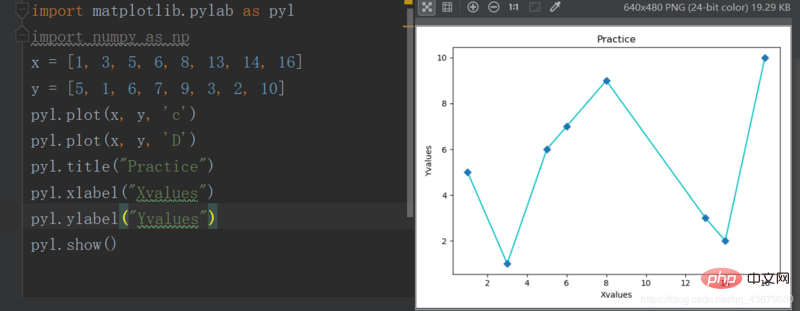6.现在绘图的x,y轴的范围是系统自动生成的，我们要想自定义，需要加上下面的两条语句，括号内为取值范围pyl.xlim()
pyl.ylim()
7.如果要在同一幅图中绘制多个图像，只需在show()之前再定义另外两个变量即可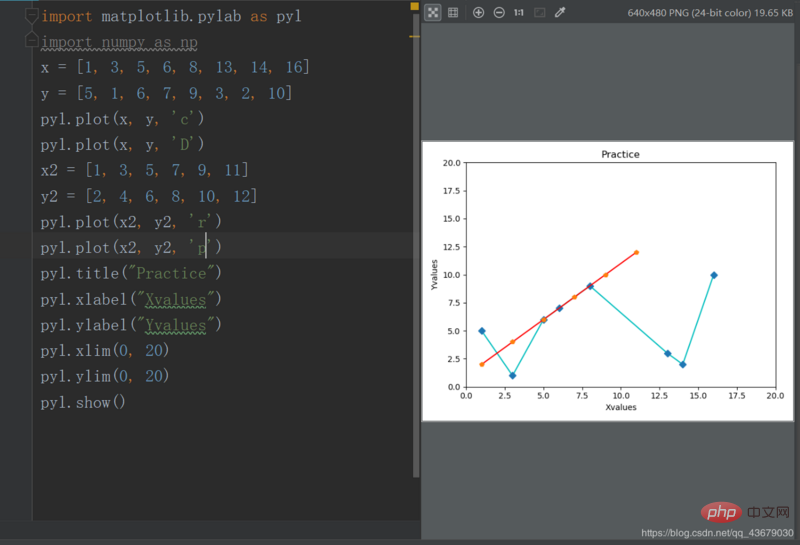以上就是Python绘制折线图和散点图的详细方法介绍（代码示例）的详细内容，更多请关注php中文网其它相关文章！
本文转载于：segmentfault，如有侵犯，请联系a@php.cn删除
展开全文• import matplotlib.pyplot as plt import numpy as np plt.rcParams['font.family'] = ['SimHei'] plt.rcParams['axes.unicode_minus'] = False n = 1024 ...plt.scatter(x,y)#绘制散点图 plt.title
import matplotlib.pyplot as plt

import numpy as np

plt.rcParams['font.family'] = ['SimHei']
plt.rcParams['axes.unicode_minus'] = False

n = 1024
x = np.random.normal(0,1,n)#生成1024个点，点的范围为0~1
y = np.random.normal(0,1,n)

plt.scatter(x,y)#绘制散点图

plt.title("绘制散点图")

plt.show()

展开全文数据可视化 可视化 深度学习
• import matplotlib.pyplot as plt plt.rcParams['font.family'] = ['SimHei'] x = [2015,2016,2017,2018,2019]#x轴坐标...plt.plot(x,y,'b',label = 'wenke',linewidth = 2)#绘制线段 plt.plot(x,y1,'m',label = 'lik
import matplotlib.pyplot as plt

plt.rcParams['font.family'] = ['SimHei']

x = [2015,2016,2017,2018,2019]#x轴坐标数据
y = [500,512,514,530,516]#y轴坐标数据
y1 = [520,521,526,545,563]
plt.plot(x,y,'b',label = 'wenke',linewidth = 2)#绘制线段
plt.plot(x,y1,'m',label = 'like',linewidth = 2)
plt.title('绘制折线图')#添加图表标题

plt.ylabel('成绩')#添加y轴标题
plt.xlabel('年份')#添加x轴标题

plt.legend()#设置图例
plt.legend()
plt.savefig('折线图',dip = 72)#以分辨率72来保存图片

plt.show()#显示图形

展开全文可视化 数据分析 数据可视化
• ## 用python绘制散点图

万次阅读 多人点赞 2018-07-13 21:41:53
今天下午学习了如何使用python绘制简单的散点图，写成博客分享一下。 在python中画散点图主要是用matplotlib模块中的scatter函数，先来看一下scatter函数的基本信息。 网址为：点击打开链接 可以看到scatter中有很多...
在python中画散点图主要是用matplotlib模块中的scatter函数，先来看一下scatter函数的基本信息。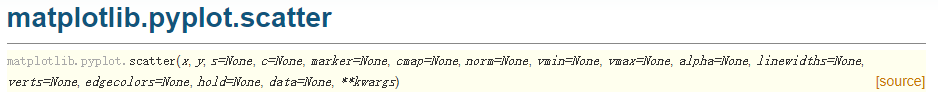网址为：点击打开链接
可以看到scatter中有很多参数，经常使用的参数主要有以下几个：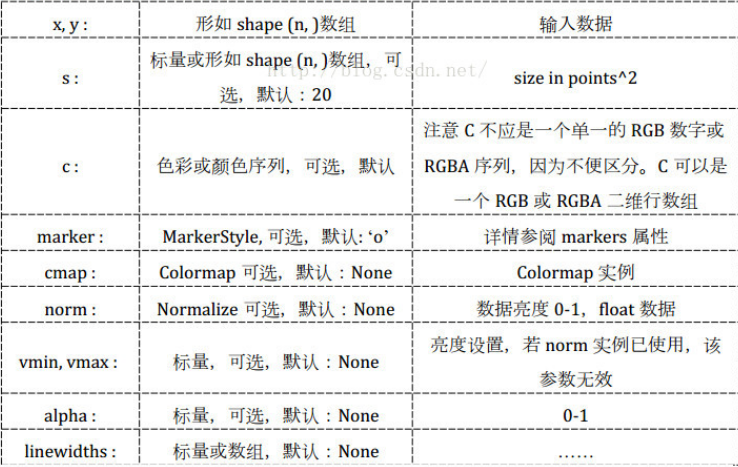c：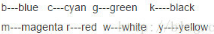marker: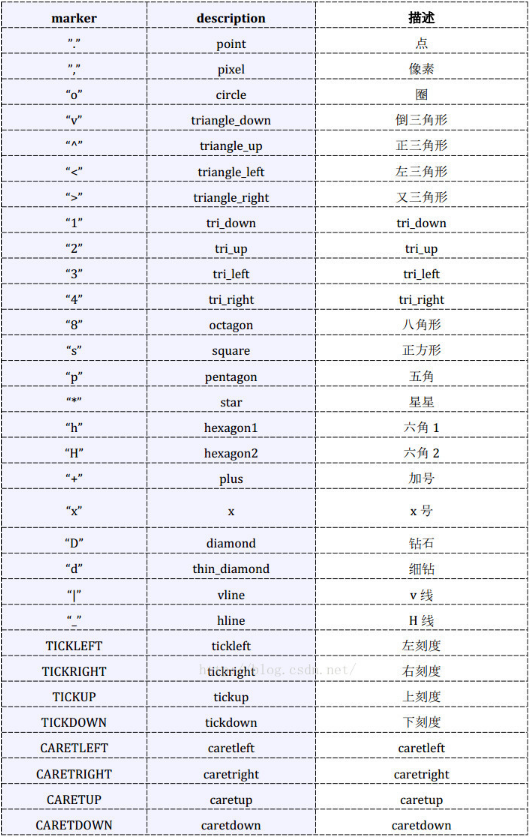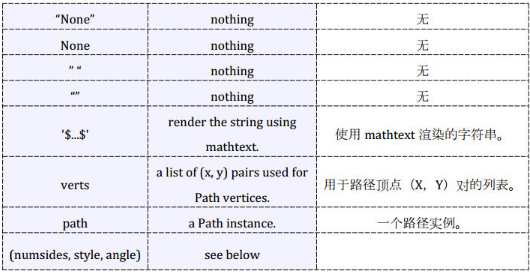数据、代码和绘制的图如下。
数据（取第一列作为x，取第四列作为y）截图：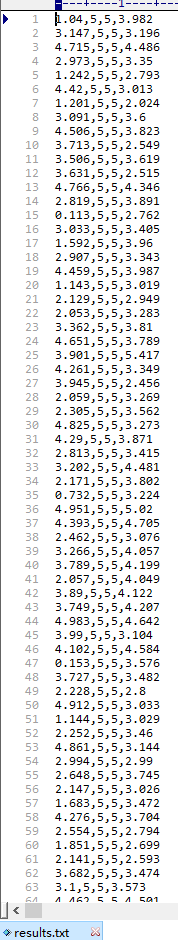代码如下：
import matplotlib.pyplot as plt
import numpy as np

# 定义画散点图的函数
def draw_scatter(n, s):
"""
:param n: 点的数量，整数
:param s:点的大小，整数
:return: None
"""
# 加载数据
# 通过切片获取横坐标x1
x1 = data[:, 0]
# 通过切片获取纵坐标R
y1 = data[:, 3]
# 横坐标x2
x2 = np.random.uniform(0, 5, n)
# 纵坐标y2
y2 = np.array( * n)
# 创建画图窗口
fig = plt.figure()
# 将画图窗口分成1行1列，选择第一块区域作子图
# 设置标题
ax1.set_title('Result Analysis')
# 设置横坐标名称
ax1.set_xlabel('gamma-value')
# 设置纵坐标名称
ax1.set_ylabel('R-value')
# 画散点图
ax1.scatter(x1, y1, s=s, c='k', marker='.')
# 画直线图
ax1.plot(x2, y2, c='b', ls='--')
# 调整横坐标的上下界
plt.xlim(xmax=5, xmin=0)
# 显示
plt.show()

# 主模块
if __name__ == "__main__":
# 运行
draw_scatter(n=2000, s=20)
绘制的图如下：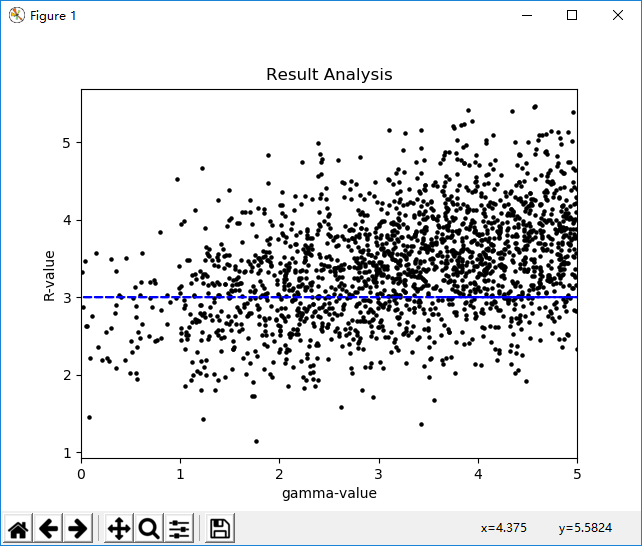展开全文scatter
• 1、示例 1 代码import numpy as npimport pandas as pdimport matplotlib.pyplot as plt# 生成数据v1 = np.random.normal(0, 1, 100)v2 = np.random.randint(0, 23, 100)v3 = v1 * v2# 3*100 的数据框df = pd....
• 本文是从matplotlib官网上摘录下来的一个实例，实现的功能是Python+matplotlib绘制自定义饼图作为散点图的标记，具体如下。首先看下演示效果实例代码：import numpy as npimport matplotlib.pyplot as plt# first ...
• python绘制散点图并标记序号的方法实现二维平面上散点的绘制，并可以给每个散点标记序号或者名称：import numpy as npimport matplotlib.pyplot as pltx=[2.3,4.5,3,7,6.5,4,5.3]y=[5,4,7,5,5.3,5.5,6.2]n=np.arange...
• 环境：windows系统，anaconda3 64位，python 3.61.初认识基本代码如下：import numpy as npimport matplotlib.pyplot as pltN = 1000x = np.random.randn(N)y = np.random.randn(N)plt.scatter(x, y)plt.show()这里...
• ## Python绘制折线图、散点图

万次阅读 多人点赞 2019-01-06 11:01:13
Python绘图需要下载安装matplotlib模块，它是一个数学绘图库，我们将使用它来制作简单的图表，如折线图散点图。关于matplotlib模块的下载安装预配置将在后面具体介绍。 （一）绘制折线图 首先，我们先绘制一个...大数据 机器学习
• 1.画最简单的直线图代码如下：import numpy as npimport matplotlib.pyplot as pltx=[0,1]y=[0,1]plt.figure()plt.plot(x,y)plt.savefig("easyplot.jpg")结果如下：代码解释：#x轴，y轴x=[0,1]y=[0,1]#...
• 代码是利用python绘制散点图，使用xlrd读取Excel文件，
• 主要介绍了python绘制简单折线图代码示例，具有一定借鉴价值，需要的朋友可以参考下。
• 今天教大家用python绘制一些线性图案，需要的朋友可以借鉴参考一下。画最简单的直线图代码如下：import numpy as npimport matplotlib.pyplot as pltx=[0,1]y=[0,1]plt.figure()plt.plot(x,y)plt.savefig("...
• In Python, with Matplotlib, how can a scatter plot with empty circles be plotted? The goal is to draw empty circles around some of the colored disks already plotted by scatter(), so as to highlight t....
• 本人在学习使用Python和plotly处理数据时，经过两个小时艰难试错，终于完成了散点图折线图的实例。在使用过程中遇到一个大坑，因为官方给出的案例是用在线存储的，所以需要安装jupyter（也就是ipython）才能使用...
• 通过python绘制散点图1）不同类别的点标注不同的颜色；2）绘制图例；具体实现需求1：将excel的数据导入python然而这一次的excel文件中数据长度不一致，python会读取长度为最长列长度（MaxRow）的所有数据，如果某些...
• 本篇文章给大家带来的内容是关于Python绘制折线图散点图的详细方法介绍（代码示例），有一定的参考价值，有需要的朋友可以参考一下，希望对你有所帮助。1、绘制折线图散点图要用到matplotlib下的pylab，因此我们...
• 与线型图类似的是，散点图也是一个个点集构成的。但不同之处在于，散点图的各点之间不会按照前后...用plt.scatter画散点图scatter专门用于绘制散点图，使用方式和plot方法类似，区别在于前者具有更高的灵活性，可以...
• 与线型图类似的是，散点图也是一个个点集构成的。但不同之处在于，散点图的各点之间不会按照前后...用plt.scatter画散点图scatter专门用于绘制散点图，使用方式和plot方法类似，区别在于前者具有更高的灵活性，可以......

# python绘制散点图的代码python 订阅